# Pandas DataFrame Plots

Pandas

Pandas.DataFrame.plot to get line graphs using data

Let us create a DataFrame with name of the students and their marks.
A series of data joined by lines to draw a line graph.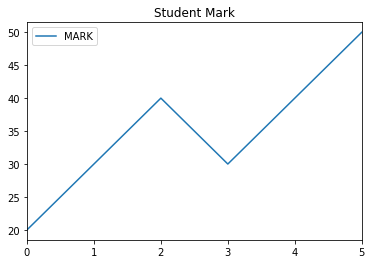Data represented by set of vertical Bars .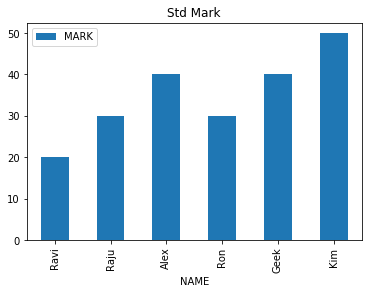Data represented by set of Horizontal Bars .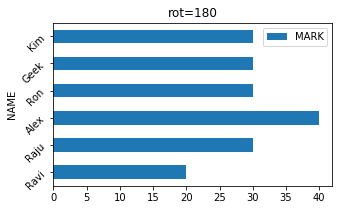distribution of numerical or categorical data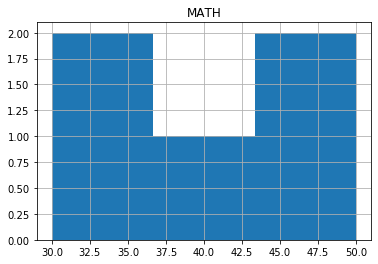depicting groups of numerical data through their quartiles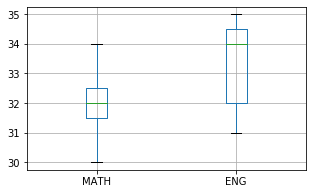Pie Chart showing relative percentage distribution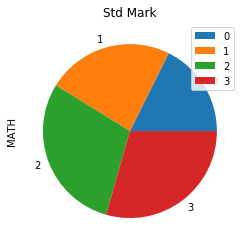distribution of a numeric variabledisplays graphically quantitative data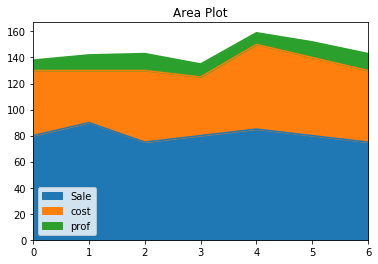values for typically two variables for a set of data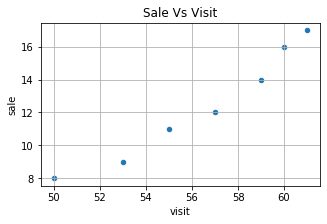relationship of 2 numerical variables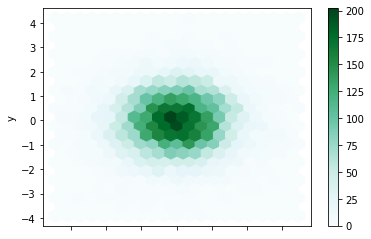## Saving graph as image

``````plot=df.plot.pie(title="Colors",y='MATH',autopct='%1.1f%%')
fig = plot.get_figure()
fig.savefig("D:\\my_data\\output2.png")``````
With `subplots=True`
``````plot=df.plot.line(subplots=True).get_figure()
plot.savefig('my_pic.jpg')``````
Plotting graphs using DataFrame
 plot-line Plotting line graph with different options plot-bar Plotting bar graph with different options plot-barh Plotting Horizontal bar graph with different options plot-hist Histogram with different options plot-boxplot boxplot with different options plot-pie Pie diagram with different options plot-density Density diagram with different options plot-area Area diagram with different options plot-scatter Scatter plot with different options

Subscribe to our YouTube Channel here

## Subscribe

* indicates required
Subscribe to plus2netplus2net.com